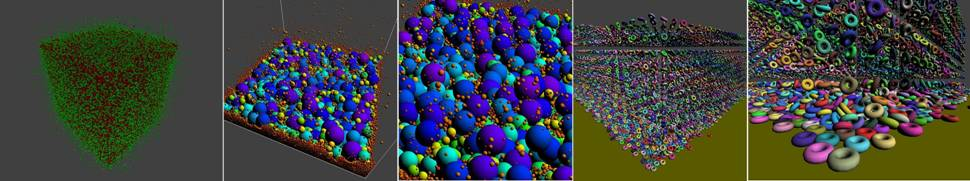Real-time Collision Culling of a Million Bodies on

Graphics Processing Units

 Fuchang Liu1,  Takahiro Harada2, Youngeun Lee1 and Young J. Kim1 1Department of Computer Science & Engineering Ewha Womans University, Seoul, Korea 2Advanced Micro Devices, Inc.

 1.   Published in the ACM Transactions on Graphics* (SIGGRAPH ASIA), Dec. 2010(pdf) 2. Source Code in C++

# Abstract

We cull collisions between very large numbers of moving bodies using graphics processing units (GPUs). To perform massively parallel sweep-and-prune (SaP), we mitigate the great density of intervals along the axis of sweep by using principal component analysis to choose the best sweep direction, together with spatial subdivisions to further reduce the number of false positive overlaps. Our algorithm implemented entirely on GPUs using the CUDA framework can handle a million moving objects at interactive rates. As application of our algorithm, we demonstrate the real-time simulation of very large numbers of particles and rigid-body dynamics.

# Benchmarking Scenarios

1. Random Configurations:

We used a benchmark setup similar to the Bullet collision Library, in which a set of AABBs are uniformly distributed in space and moving randomly. As we changed the number of AABBs from 16K to 960K, we measured the performance of our algorithm and that of the three broad-phase CD algorithms provided in Bullet, which are BoxPruning, ArraySaP and an AABB dynamic tree. The first two algorithms are based on SaP and the last one using a dynamic bounding volume hierarchy. All of these algorithms run on CPU. The size of the AABBs also varies from 0.5% to 8% to the size of the bounding box of the workspace. As shown Figure below, our algorithm outperforms the fastest of the Bullet implementations (i.e. the AABB dynamic tree) by a factor of 71 times.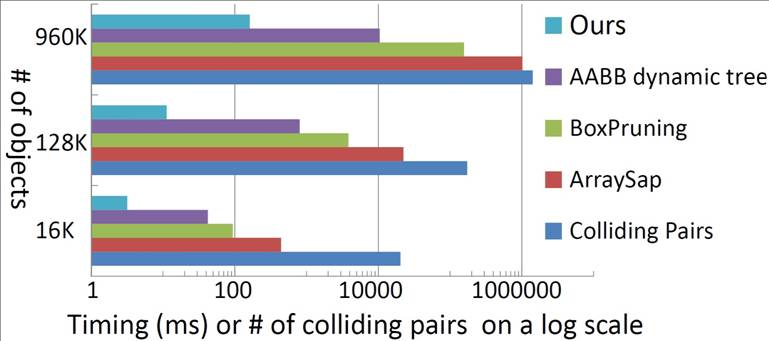Comparison of Collision Detection performance with the difference methods in Bullet CPU algorithm   We also investigated the the performance of our algorithm when only some of the objects are moving. The objects are one million AABBs of varying sizes, and we changed the percentage of moving objects from 5% to 25%. The figure below shows that the number of new collisions generated by moving objects, as a proportion of the total number of interferences is almost linear with computation time, which implies that as more collision pairs are introduced by moving objects, our algorithm requires more time to process them. This means that our algorithm efficiently utilizes the collision results introduced by static objects, which are cached from the previous time step.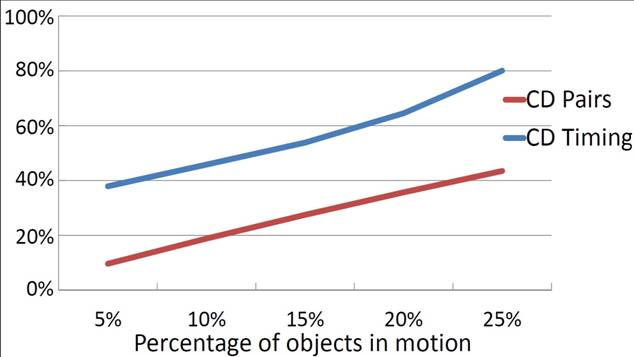Collision detection when only some objects are moving

2. Particle Simulation:

We benchmarked on large sets of particles of varying sizes. We did this by modifying an open particle simulation demo, originally from NVIDIA (Particles sample code in CUDA SDK). As shown in Figure below, we introduced 100K and 0.3M spheres of the size varying from 0.3% to 20% of the dimension of the workspace and simulated their motions under gravity. We then measure the performance of our algorithm and that of a uniform subdivision algorithm that also runs GPUs. While CD takes up most of the computation, it is hard to decouple the collision times from the simulation times using NVIDIA's uniform subdivision method. However, for 100K and 0.3M particles, our algorithm takes 56 ms and 252 ms on average for both collision detection and particle simulation while uniform subdivision 4452 ms and 53464 ms; thus our algorithm outperforms uniform subdivision by a factor of 212 times.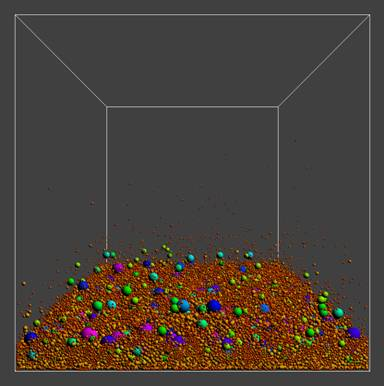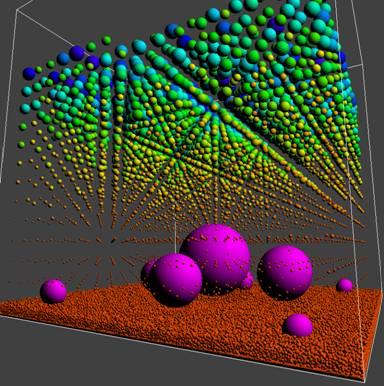Particle Simulation

3. Approximate Rigid-Body Dynamics:

We approximated a rigid model with a set of uniform spheres, and used a penalty-based approach running in parallel on GPUs.  This avoids narrow-phase Collision Detection. We simulated 16K torus models approximated by six spheres of varying size moving under gravity. We were able to simulate the approximate rigid-body dynamics entirely running on GPUs in 18 ms, including collision detection.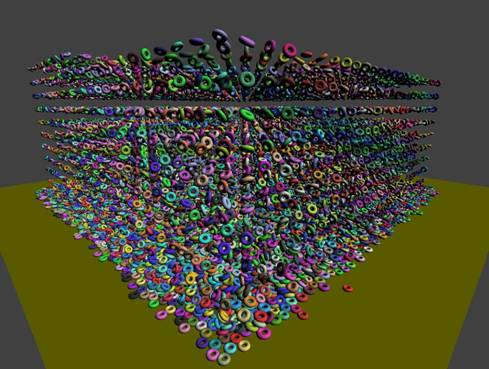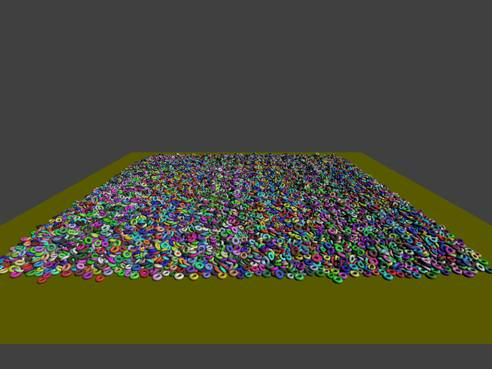Approximate Rigid-Body Dynamics for torus